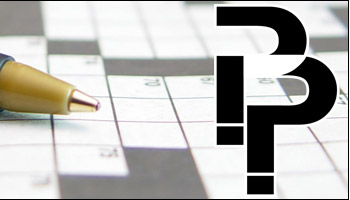# Puzzle Ideas - Codeword puzzles

## Codeword puzzles - crack the code and solve the puzzle

codeword, codeword puzzles, code, crosswords, puzzles, logic puzzles, number puzzles,

# Codeword puzzles# How to solve a codeword puzzle

In a codeword puzzle, each letter used in the puzzle is assigned a number, and when the puzzle is printed, this number is inserted into the cell instead of the letter. To complete the puzzle, the solver must work out which letter corresponds to each of the numbers. A Scratch Pad is printed along with the puzzle and can be used by the solver to record the best guess as to what this relationship might be. Similarly, a Used Letter table can be used to record which letters have been used so far in the solution.

Puzzle Ideas - Codeword puzzles

Codeword puzzles - crack the code and solve the puzzle

codeword, codeword puzzles, code, crosswords, puzzles, logic puzzles, number puzzles,